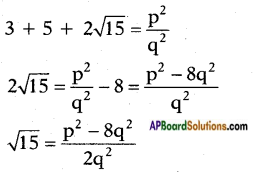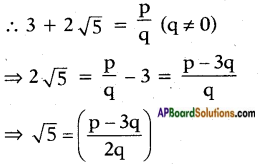# AP SSC 10th Class Maths Solutions Chapter 1 Real Numbers Ex 1.4

AP State Board Syllabus AP SSC 10th Class Maths Textbook Solutions Chapter 1 Real Numbers Ex 1.4 Textbook Questions and Answers.

## AP State Syllabus SSC 10th Class Maths Solutions 1st Lesson Real Numbers Exercise 1.4

### 10th Class Maths 1st Lesson Real Numbers Ex 1.4 Textbook Questions and Answers

Question 1.
Prove that the following are irrational,
i) $$\frac{1}{\sqrt{2}}$$
ii) √3 + √5
iii) 6 + √2
iv) √5
v) 3 + 2√5
i) $$\frac{1}{\sqrt{2}}$$
On the contrary, suppose that is a $$\frac{1}{\sqrt{2}}$$ rational number;
then $$\frac{1}{\sqrt{2}}$$ is of the form where $$\frac{p}{q}$$ and q are integers.
∴ $$\frac{1}{\sqrt{2}}$$ = $$\frac{p}{q}$$
⇒ $$\frac{\sqrt{2}}{1}$$ = $$\frac{q}{p}$$
(i.e.,) √2 is a rational number and it is a contradiction. This contradiction arised due to our supposition that $$\frac{1}{\sqrt{2}}$$ is a rational number.
Hence $$\frac{1}{\sqrt{2}}$$ is an irrational number.ii) Suppose √3 + √5 is not an irrational number.
Then √3 + √5 must be a rational number.
√3 + √5 = $$\frac{p}{q}$$, q ≠ 0 and p, q ∈ Z
Squaring on both sidesbut √15 is an irrational number.
$$\frac{p^{2}-8 q^{2}}{2 q^{2}}$$ is a rational number
(p2 – 8q2, 2q2 ∈ Z, 2q2 ≠ 0)
but an irrational number can’t be equal to a rational number, so our supposition that √3 + √5 is not an irrational number is false.
∴ √3 + √5 is an irrational number.

iii) 6 + √2
To prove: 6 + √2 is an irrational number.
Let us suppose that 6 + √2 is a rational number.
∴ 6 + √2 = $$\frac{p}{q}$$, q ≠ 0
⇒ √2 = $$\frac{p}{q}$$ – 6
⇒ √2 = Difference of two rational numbers
⇒ √2 = rational number But this contradicts the fact that √2 is an irrational number.
∴ Our supposition is wrong.
Hence the given statement is true.
6 + √2 is an irrational number.

iv) √5
To prove: √5 is an irrational number.
On the contrary, let us assume that √5 is a rational number.
∴ √5 = $$\frac{p}{q}$$, q ≠ 0
If p, q have a common factor, on cancelling the common factor let it be
reduces to $$\frac{a}{b}$$ where a, b are co-primes.
Now √5 = $$\frac{a}{b}$$, where HCF (a, b) = 1
Squaring on both sides we get
⇒ (√5)2 = $$\left(\frac{a}{b}\right)^{2}$$
⇒ 5 = $$\frac{\mathrm{a}^{2}}{\mathrm{~b}^{2}}$$
⇒ 5b2 = a2
⇒ 5 divides a2 and thereby 5 divides 5
Now, take a = 5c
then, a2 = 25c2
i.e., 5b2 = 25c2
⇒ b2 = 5c2
⇒ 5 divides b2 and thereby b.
⇒ 5 divides both b and a.
This contradicts that a and b are co-primes.
This contradiction arised due to our assumption that √5 is a rational number.
Hence our assumption is wrong and the given statement is true, i.e., √5 is an irrational number,v) 3 + 2√5
To Prove: 3 + 2√5 is an irrational.
On the contrary, let us assume that 3 + 2√5 is a rational number.Here p, q being integers we can say that $$\frac{p-3q}{2q}$$ is a rational number.
This contradicts the fact that √5 is an irrational number. This is due to our assumption “3 + 2√5 is a rational number”.
Hence our assumption is wrong.
∴ 3 + 2√5 is an irrational number.

Question 2.
Prove that √p + √q is an irrational, where p, q are primes.